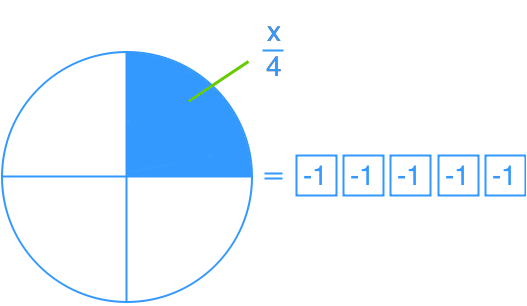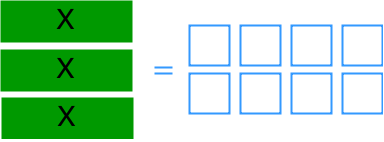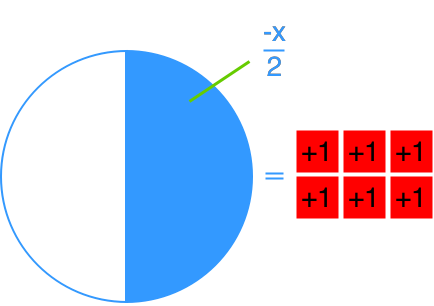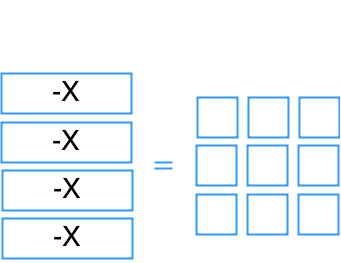# Model and solve one-step linear equations: $ax = b$, $\frac{x}{a} = b$

### Model and solve one-step linear equations: $ax = b$, $\frac{x}{a} = b$

Linear equations which can be solved with a single operation are called one-step linear equations. In this section, we will try to solve one-step linear equations represented in diagrams and in equation form.

#### Lessons

• Introduction
a)
What is Reflexive property of equality?

b)
What is Transitive property of equality?

c)
What is Substitution property of equality?

d)
What is Symmetric property of equality?

e)
How to solve one-step equations?

• 1.
What is the equation represented by each diagram?
a)b)c)d)• 2.
Solve.
a)
$- 4x = 56$

b)
$3x = - 24$

c)
$\frac{x}{5} = - 75$

d)
$- 2 = \frac{x}{{ - 10}}$

• 3.
Bill is able to type 35 words every minute. If he has typed 385 words so far, how many minutes has he been typing for?

• 4.
Suzy and 2 friends split their restaurant bill evenly. Each person paid \$16. How much did the bill cost?